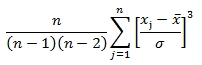# SQL Server SKEW function

SKEW

Updated: 30 September 2010

Note: This documentation is for the SQL2008 (and later) version of this XLeratorDB function, it is not compatible with SQL Server 2005.

Use the multi-input aggregate function SKEW to return the degree of asymmetry of a distribution. If the distribution has a longer tail less than the maximum, the function has negative skewness. Otherwise it has positive skewness. The equation for skewness is:Syntax
SELECT [wctStatistics].[wct].[SKEW] (
<@Kown_x, float,>
Arguments
@Known_x
the x-values to be used in the SKEW calculation. @Known_x is an expression of type float or of a type that can be implicitly converted to float.
Return Types
float
Remarks
·         If there are fewer than three rows in the dataset, then SKEW returns a NULL.
·         If the sample standard deviation is zero, then SKEW returns a NULL.
·         RSQ is an AGGREGATE function and follows the same conventions as all other AGGREGATE functions in SQL Server.

Examples
In this example, we calculate the SKEW for a single set of x-values
SELECT wct.SKEW(x) AS SKEW
FROM (
SELECT 6.1 UNION ALL
SELECT -4.2 UNION ALL
SELECT -10 UNION ALL
SELECT 9.6 UNION ALL
SELECT 9.6 UNION ALL
SELECT 4.3 UNION ALL
SELECT 2.3 UNION ALL
SELECT 7.8 UNION ALL
SELECT -7.8 UNION ALL
SELECT 7.2
) n(x)

This produces the following result
SKEW
----------------------
-0.838669119197463

(1 row(s) affected)
In this example, we will populate some temporary table with some information about male height at some selected colleges and then calculate the SKEW value. First, create the table and put some data in it:
CREATE TABLE #c(
College           nvarchar(50),
Mark        bigint,
Freq        bigint
)

INSERT INTO #c VALUES ('Faber College',61,5)
INSERT INTO #c VALUES ('Faber College',64,18)
INSERT INTO #c VALUES ('Faber College',67,42)
INSERT INTO #c VALUES ('Faber College',70,27)
INSERT INTO #c VALUES ('Faber College',73,7)
INSERT INTO #c VALUES ('Faber College',76,1)
INSERT INTO #c VALUES ('Faber College',79,0)
INSERT INTO #c VALUES ('Harrison University',61,4)
INSERT INTO #c VALUES ('Harrison University',64,23)
INSERT INTO #c VALUES ('Harrison University',67,36)
INSERT INTO #c VALUES ('Harrison University',70,27)
INSERT INTO #c VALUES ('Harrison University',73,8)
INSERT INTO #c VALUES ('Harrison University',76,1)
INSERT INTO #c VALUES ('Harrison University',79,1)
INSERT INTO #c VALUES ('Adams College',61,4)
INSERT INTO #c VALUES ('Adams College',64,24)
INSERT INTO #c VALUES ('Adams College',67,42)
INSERT INTO #c VALUES ('Adams College',70,17)
INSERT INTO #c VALUES ('Adams College',73,11)
INSERT INTO #c VALUES ('Adams College',76,2)
INSERT INTO #c VALUES ('Adams College',79,0)
INSERT INTO #c VALUES ('Western University',61,7)
INSERT INTO #c VALUES ('Western University',64,11)
INSERT INTO #c VALUES ('Western University',67,51)
INSERT INTO #c VALUES ('Western University',70,29)
INSERT INTO #c VALUES ('Western University',73,1)
INSERT INTO #c VALUES ('Western University',76,0)
INSERT INTO #c VALUES ('Western University',79,1)
INSERT INTO #c VALUES ('Jordan College',61,11)
INSERT INTO #c VALUES ('Jordan College',64,22)
INSERT INTO #c VALUES ('Jordan College',67,46)
INSERT INTO #c VALUES ('Jordan College',70,18)
INSERT INTO #c VALUES ('Jordan College',73,3)
INSERT INTO #c VALUES ('Jordan College',76,0)
INSERT INTO #c VALUES ('Jordan College',79,0)
Now, calculate the SKEW value of the height distribution
SELECT wct.SKEW(Freq) as SKEW
FROM #c
This returns the following result
SKEW
----------------------
0.982741176782763

(1 row(s) affected)
If we wanted to calculate the SKEW by college, we would enter the following statement.
SELECT College
,wct.SKEW(Freq) as SKEW
FROM #c
GROUP BY college
This returns the following result
College                                            SKEW
-------------------------------------------------- ----------------------
Faber College                                      1.03800625232578
Harrison University                                0.566337936272629
Jordan College                                     1.313727662056
Western University                                 1.49860523896341

(5 row(s) affected)
If we only wanted to return results not between -1 and 1, then we could enter the following statement.
SELECT College
,wct.SKEW(Freq) as SKEW
FROM #c
GROUP BY college
HAVING ABS(wct.SKEW(Freq)) > 1
This returns the following result
College                                            SKEW
-------------------------------------------------- ----------------------
Faber College                                      1.03800625232578
Jordan College                                     1.313727662056
Western University                                 1.49860523896341

(4 row(s) affected)

### SupportCopyright 2008-2021 Westclintech LLC         Privacy Policy        Terms of Service